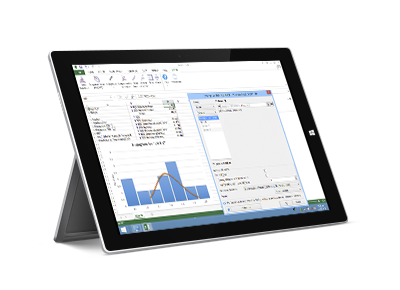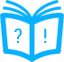# StatPlus for Windows

With StatPlus, one gets a robust suite of statistics tools and graphical analysis methods that are easily accessed through a simple and straightforward interface. The range of possible applications of StatPlus is virtually unlimited - sociology, financial analysis, biostatistics, economics, insurance industry, healthcare and clinical research - to name just a few fields where the program is already being extensively used.

While StatPlus is a professional statistical analysis tool, the interface is so simple that even people who have no knowledge of statistics are capable of processing data, provided they know how to use PC and clear instructions are given. This frees up intellectual resources for analyzing the results, rather than agonizing over who and how processed the data, and if any mistakes were made in the process.Standalone version reads numerous text formats, Microsoft* Excel* 97-2003 (XLS) and 2007-2021 (XLSX) workbooks, SPSS* Documents and supports almost all Excel built-in worksheet functions (math, statistical, financial). StatPlus comes with Excel add-in (StatFi) that transforms Excel into a statistical software package.

### Statistics A-Z

StatPlus allows to perform various forms of analysis - from data transformation and sampling to complex analysis, including as non-parametric and regression analysis, survival analysis, and a wide variety of other methods.

View Statistical Features List

### Multi-platform

StatPlus is available for both PC and Mac platforms at no extra charge. Both versions include standalone spreadsheet and Excel add-in. Save learning time and costs for your mixed PC and Mac environment.

Looking for Mac version?

### Free Trial

We have free trial that gives you an opportunity to evaluate the software before you purchase it. Should you have any questions during the trial period, please feel free to contact our Support Team.

### Affordable

You will benefit from the reduced learning curve and attractive pricing while enjoying the benefits of precise routines and calculations. Mac/PC license is permanent, there is no renewal charges.

Get a Quote Today

### Requirements

StatPlus requires Windows 2000 or newer, Windows 8 or newer recommended. Excel add-in (StatFi) requires Excel 2007 or newer. StatPlus supports Windows 11 and Excel 2021.### StatPlus and StatFi Features List

• Pro Features
• Fast and powerful standalone spreadsheet.
• Add-in for Excel 2007, 2010, 2013, 2016, 2019 and 2021.
• Priority support.
• Options to emulate Excel Analysis ToolPak results and migration guide for users switching from Analysis ToolPak.
• Basic Statistics
• Detailed descriptive statistics.
• One-sample t-test.
• Two-sample t-test.
• Two-sample t-test for summarized data.
• Fisher F-test.
• One-sample and two-sample z-tests.
• Correlation analysis and covariance.
• Normality tests (Jarque-Bera, Shapiro-Wilk, Shapiro-Francia, Cramer-von Mises, Anderson-Darling, Kolmogorov-Smirnov, D'Agostino's tests).
• Cross-tabulation and Chi-square.
• Frequency tables analysis (for discrete and continuous variables).
• Multiple definitions for computing quantile statistics.
• Analysis of Variance (ANOVA)
• One-way and two-way ANOVA (with and without replications).
• Three-way analysis of variance.
• Post-hoc comparisons - Bonferroni, Tukey-Kramer, Tukey B, Tukey HSD, Neuman-Keuls, Dunnett.
• General Linear Models (GLM) ANOVA.
• Within subjects ANOVA and mixed models.
• Multivariate Analysis
• Principal component analysis (PCA).
• Factor analysis (FA).
• Discriminant function analysis.
• Hierarchical Clustering and K-Means.
• Nonparametric Statistics
• 2x2 tables analysis (Chi-square, Yates Chi-square, Exact Fisher Test, etc.).
• Rank and percentile.
• Chi-square test.
• Rank correlations (Kendall Tau, Spearman R, Gamma, Fechner).
• Comparing independent samples
Mann-Whitney U Test, Kolmogorov-Smirnov test, Wald-Wolfowitz Runs Test, Rosenbaum Criterion. Kruskal-Wallis ANOVA and Median test.
• Comparing dependent samples
Wilcoxon Matched Pairs Test, Sign Test, Friedman ANOVA, Kendall's W (coefficient of concordance).
• Cochran's Q Test.
• Regression Analysis
• Multivariate linear regression (residuals analysis, collinearity diagnostics, confidence and prediction bands).
• Weighted least squares (WLS) regression.
• Logistic regression.
• Stepwise (forward and backward) regression.
• Polynomial regression.
• Curve fitting.
• Tests for heteroscedasticity: Breusch–Pagan test (BPG), Harvey test, Glejser test, Engle's ARCH test (Lagrange multiplier) and White test.
• Time Series Analysis
• Data processing.
• Fourier analysis.
• Smoothing.
• Moving average.
• Analysis.
• Autocorrelation (ACF and PACF).
• Interrupted time series analysis.
• Unit root tests - Dickey–Fuller, Augmented Dickey–Fuller (ADF test), Phillips–Perron (PP test), Kwiatkowski–Phillips–Schmidt–Shin (KPSS test).
• Survival Analysis
• Life tables.
• Kaplan-Meier (log rank test, hazard ratios).
• Cox proportional-hazards regression.
• Probit-analysis (Finney and LPM).
LD values (LD50/ED50 and others), cumulative coefficient calculation.
• Receiver operating characteristic curves analysis (ROC analysis).
AUC methods - DeLong's, Hanley and McNeil's. Report includes: AUC (with confidence intervals), curve coordinates, performance indicators - sensitivity and specificity (with confidence intervals), accuracy, positive and negative predictive values, Youden's J (Youden's index), Precision-Recall plot.
• Comparing ROC curves.
• Data Processing
• Sampling (random, periodic, conditional).
• Random numbers generation.
• Standardization.
• Stack/unstack operations.
• Matrix operations.
• Statistical Charts
• Histogram
• Scatterplot.
• Box plot.
• Stem-and-leaf plot.
• Bland-Altman plot.
• Bland-Altman plot with multiple measurements per subject.
• Quantile-quantile Q-Q plots for different distributions.
• Control charts - X-bar, R-chart, S-chart, IMR-chart, P-chart, C-chart, U-chart, CUSUM-chart.